Example for Data Analysis

The liver package contains a collection of helper functions that make various techniques from data science more user-friendly for non-experts.

Here is an example to show how to use the functinality of the package by using the churn dataset which is available in the package.

data( churn )        # load the 'churn' dataset

str( churn )
## 'data.frame':    5000 obs. of  20 variables:
##  $state : Factor w/ 51 levels "AK","AL","AR",..: 17 36 32 36 37 2 20 25 19 50 ... ##$ area.code     : Factor w/ 3 levels "area_code_408",..: 2 2 2 1 2 3 3 2 1 2 ...
##  $account.length: int 128 107 137 84 75 118 121 147 117 141 ... ##$ voice.plan    : Factor w/ 2 levels "yes","no": 1 1 2 2 2 2 1 2 2 1 ...
##  $voice.messages: int 25 26 0 0 0 0 24 0 0 37 ... ##$ intl.plan     : Factor w/ 2 levels "yes","no": 2 2 2 1 1 1 2 1 2 1 ...
##  $intl.mins : num 10 13.7 12.2 6.6 10.1 6.3 7.5 7.1 8.7 11.2 ... ##$ intl.calls    : int  3 3 5 7 3 6 7 6 4 5 ...
##  $intl.charge : num 2.7 3.7 3.29 1.78 2.73 1.7 2.03 1.92 2.35 3.02 ... ##$ day.mins      : num  265 162 243 299 167 ...
##  $day.calls : int 110 123 114 71 113 98 88 79 97 84 ... ##$ day.charge    : num  45.1 27.5 41.4 50.9 28.3 ...
##  $eve.mins : num 197.4 195.5 121.2 61.9 148.3 ... ##$ eve.calls     : int  99 103 110 88 122 101 108 94 80 111 ...
##  $eve.charge : num 16.78 16.62 10.3 5.26 12.61 ... ##$ night.mins    : num  245 254 163 197 187 ...
##  $night.calls : int 91 103 104 89 121 118 118 96 90 97 ... ##$ night.charge  : num  11.01 11.45 7.32 8.86 8.41 ...
##  $customer.calls: int 1 1 0 2 3 0 3 0 1 0 ... ##$ churn         : Factor w/ 2 levels "yes","no": 2 2 2 2 2 2 2 2 2 2 ...

It shows that the ‘churn’ dataset as a data.frame has 20 variables and 5000 observations.

Partitioning the dataset

We partition the churn dataset randomly into two groups: train set (80%) and test set (20%). Here, we use the partition function from the liver package:

set.seed( 5 )

data_sets = partition( data = churn, prob = c( 0.8, 0.2 ) )

train_set = data_sets $part1 test_set = data_sets$ part2

actual_test  = test_set \$ churn

Classification by kNN algorithm

The churn dataset has 19 predictors along with the target variable churn. Here we use the following predictors:

account.length, voice.plan, voice.messages, intl.plan, intl.mins, day.mins, eve.mins, night.mins, and customer.calls.

First, based on the above predictors, find the k-nearest neighbor for the test set, based on the training dataset, for the k = 8 as follows

formula = churn ~ account.length + voice.plan + voice.messages + intl.plan + intl.mins +
day.mins + eve.mins + night.mins + customer.calls

predict_knn = kNN( formula, train = train_set, test = test_set, k = 8 )

To report Confusion Matrix:

conf.mat( predict_knn, actual_test )
##           Actual
## Prediction yes  no
##        yes  43   7
##        no   92 882
conf.mat.plot( predict_knn, actual_test )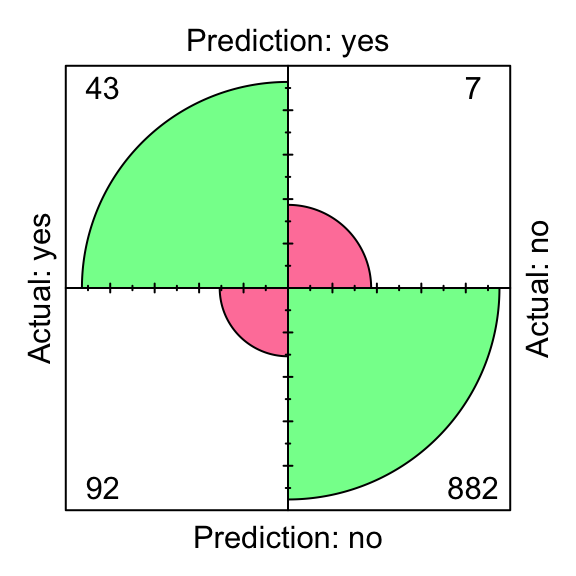To report Mean Squared Error (MSE):

mse( predict_knn, actual_test )
##  0.09667969

Classification by kNN algorithm with data transformation

The predictors that we used in the previous part, do not have the same scale. For example, variable day.mins change between 0 and 351.5, whereas variable voice.plan is binary. In this case, the values of variable day.mins will overwhelm the contribution of voice.plan. To avoid this situation we use normalization. So, we use min-max normalization and transfer the predictors as follows:

predict_knn_trans = kNN( formula, train = train_set, test = test_set, k = 8, transform = "minmax" )

To report Confusion Matrix:

conf.mat.plot( predict_knn_trans, actual_test )
conf.mat.plot( predict_knn, actual_test )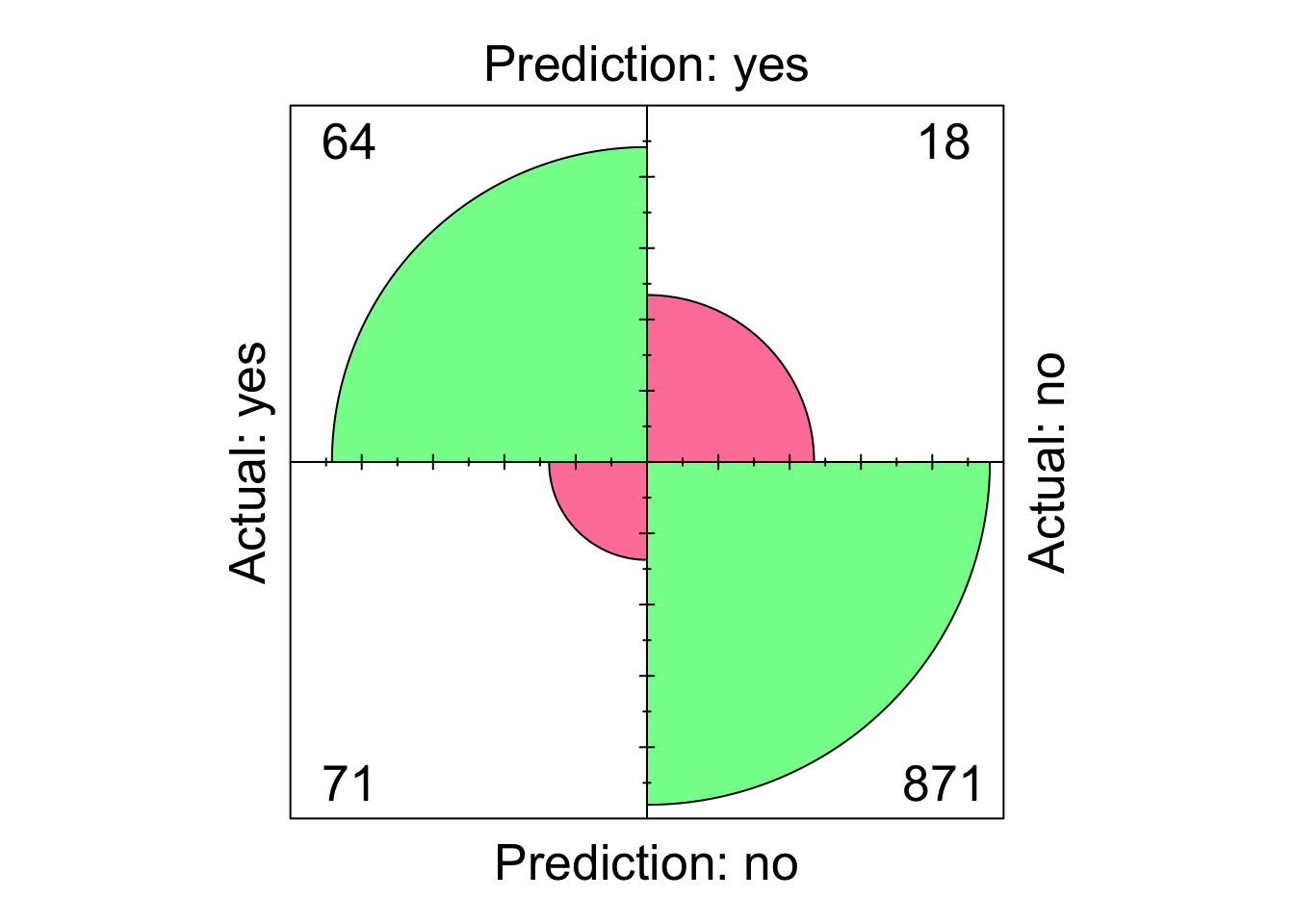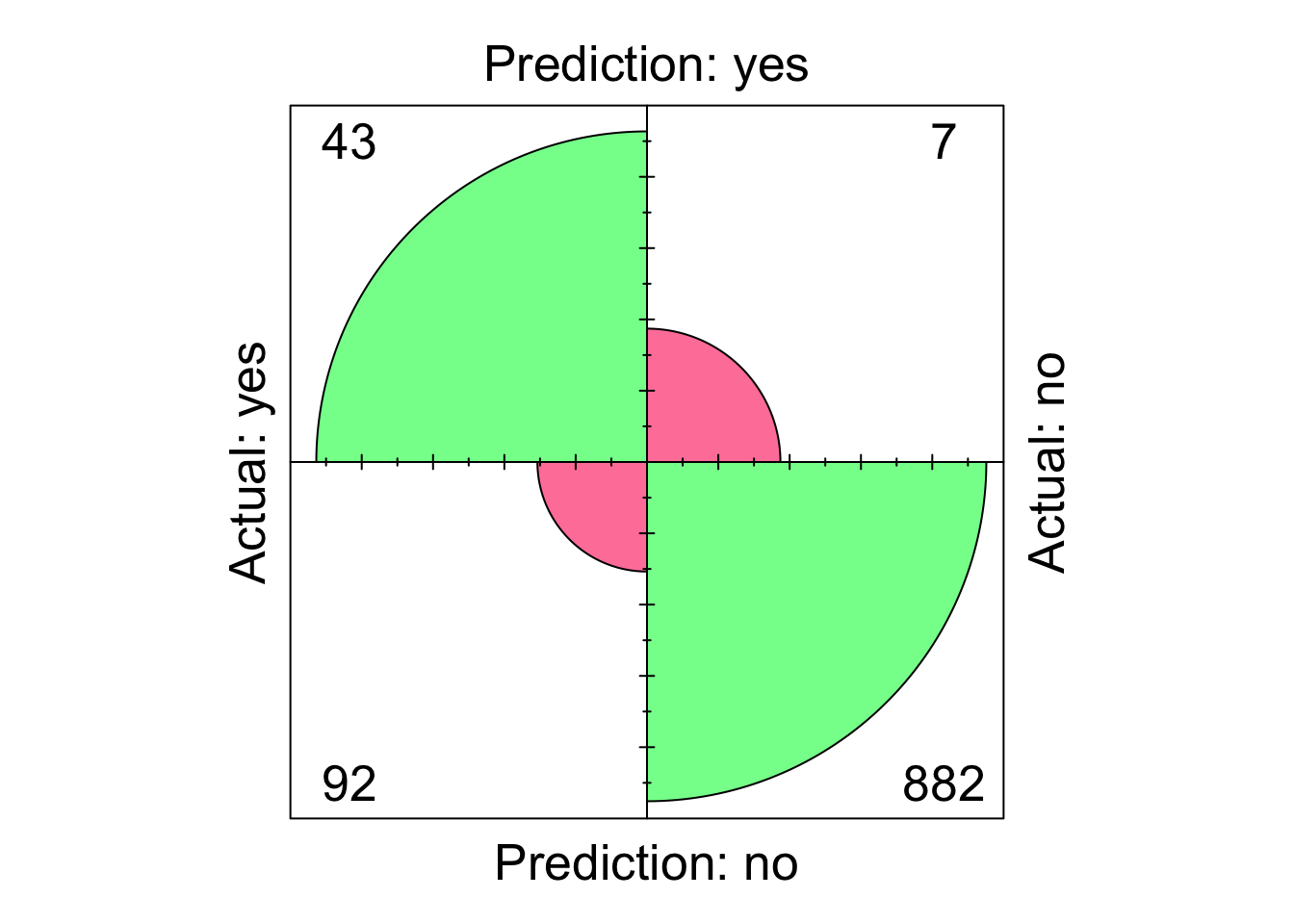To report the ROC curve, we need the probability of our classification prediction. We can have it by using:

prob_knn = kNN( formula, train = train_set, test = test_set, k = 8, type = "prob" )[ , 1 ]

prob_knn_trans = kNN( formula, train = train_set, test = test_set, transform = "minmax", k = 8, type = "prob" )[ , 1 ]

To visualize the model performance between the raw data and the transformed data, we could report the ROC curve plot as well as AUC (Area Under the Curve) by using the plot.roc function from the pROC package:

roc_knn = roc( actual_test, prob_knn )
roc_knn_trans = roc( actual_test, prob_knn_trans )

ggroc( list( roc_knn, roc_knn_trans ), size = 0.8 ) +
theme_minimal() + ggtitle( "ROC plots with AUC") +
scale_color_manual( values = c( "red", "blue" ),
labels = c( paste( "AUC=", round( auc( roc_knn ), 3 ), "; Raw data; " ),
paste( "AUC=", round( auc( roc_knn_trans ), 3 ), "; Transformed data" ) ) ) +
theme( legend.title = element_blank() ) +
theme( legend.position = c( .7, .3 ), text = element_text( size = 17 ) ) +
geom_segment( aes( x = 1, xend = 0, y = 0, yend = 1 ), color = "grey", linetype = "dashed" )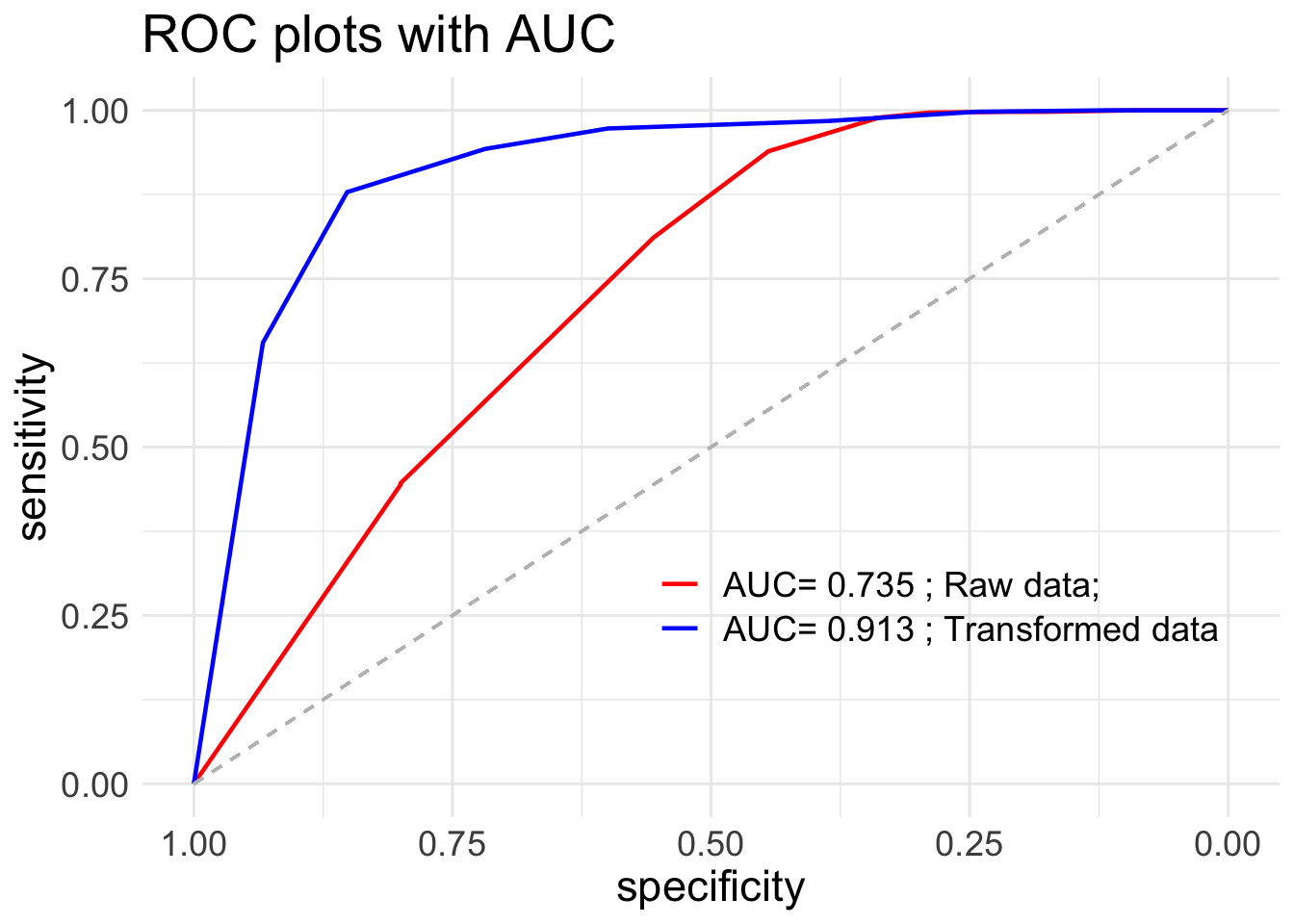Optimal value of k for the kNN algorithm

To find out the optimal value of k based on Error Rate, for the different values of k from 1 to 30, we run the k-nearest neighbor for the test set and compute the Error Rate for these models, by running kNN.plot() command

kNN.plot( formula, train = train_set, test = test_set, transform = "minmax",
k.max = 30, set.seed = 3 )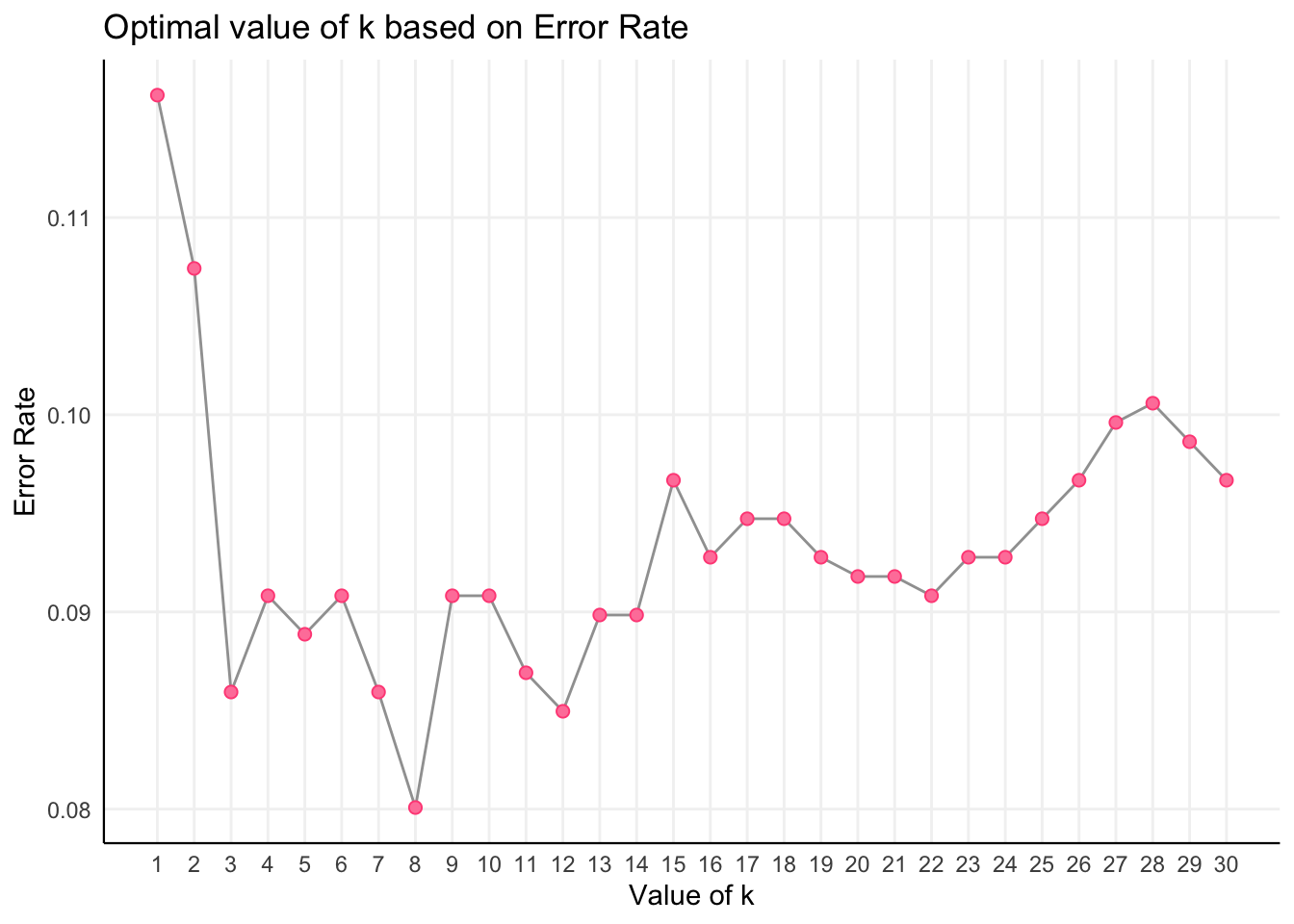The plot shows that the minimum value of Error Rate is for the case that k is 13; the smaller values of Error Rate indicates better predictions.